• ## 重投影误差

万次阅读 2016-11-30 09:44:49
重投影误差     偶尔在一篇文章中看到有关于重投影误差的介绍，简洁明了，现整理如下，同时也算是对自己图像基础知识的夯实打牢。     在计算机视觉中，经常会用到重投影误差（Reprojection error）。比如...
#重投影误差
偶尔在一篇文章中看到有关于重投影误差的介绍，简洁明了，现整理如下，同时也算是对自己图像基础知识的夯实打牢。
在计算机视觉中，经常会用到重投影误差（Reprojection error）。比如在计算平面单应矩阵和投影矩阵的时候，往往会使用重投影误差来构造代价函数，然后最小化这个代价函数，以优化单应矩阵或者投影矩阵。之所以使用重投影误差，是因为它不光考虑了单应矩阵的计算误差，也考虑了图像点的测量误差，所以其精度比单边或者对称变换误差高。
以平面单应矩阵的计算为例，假设两幅图像中的对应点满足：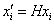其中，H是平面单应矩阵，x和x’是图像中的对应点，则重投影误差的形式如下：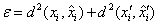subject to: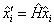其中，x是x的估计值，H是H的估计。最小化重投影误差就是优化H和x。从重投影误差公式可以看出，模型认为测量点并非绝对精确，而是存在一定的测量误差，因此需要重新估计图像点的坐标，而估计得到的新的图像点之间完美的满足单应关系。下图就是重投影误差的几何表述，d和d’的和即为重投影误差：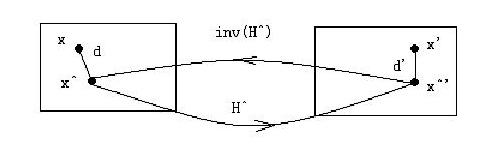上面都是在假设图像点有测量误差的情况下讨论的，如果x和x’都是图像上的点，那么确实是满足上面的模型的。但是有一种特殊情形，那就是摄像机定位时通常都会遇见的，x不是图像点，而是平面标志点在世界坐标系下的坐标，只有x’是图像点。在这种情况下，由于标志点通常都是很标准的，都是严格按尺寸制定的，所以我们可以认为x是绝对准确的，那么此时只有x’存在测量误差。如下图：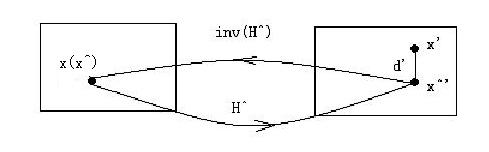此时，x的估计x就是它本身，即x=x，所以有：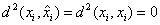那么重投影误差在强约束条件x=x^下就退化为：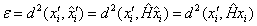上式即为单边变换误差（Transfer error）。
所以在这种情况下，单边几何变换误差才好的代价函数。也就是说，如果给重投影误差加上约束条件x=x^，重投影误差就退化为单边变换误差，并且极大地简化了优化算法。
同时，从上面的推导也可以得出结论：在使用已知标志点给摄像机定位时，重投影误差并非最好的选择，因为重投影误差模型会认为标志点存在误差，从而重新估计标志点的坐标，引入多余的误差；而此时，事实上，重投影误差已经退化为单边几何变换误差，所以在这种情况下，单边几何变换误差才好的代价函数。
再谈重投影误差：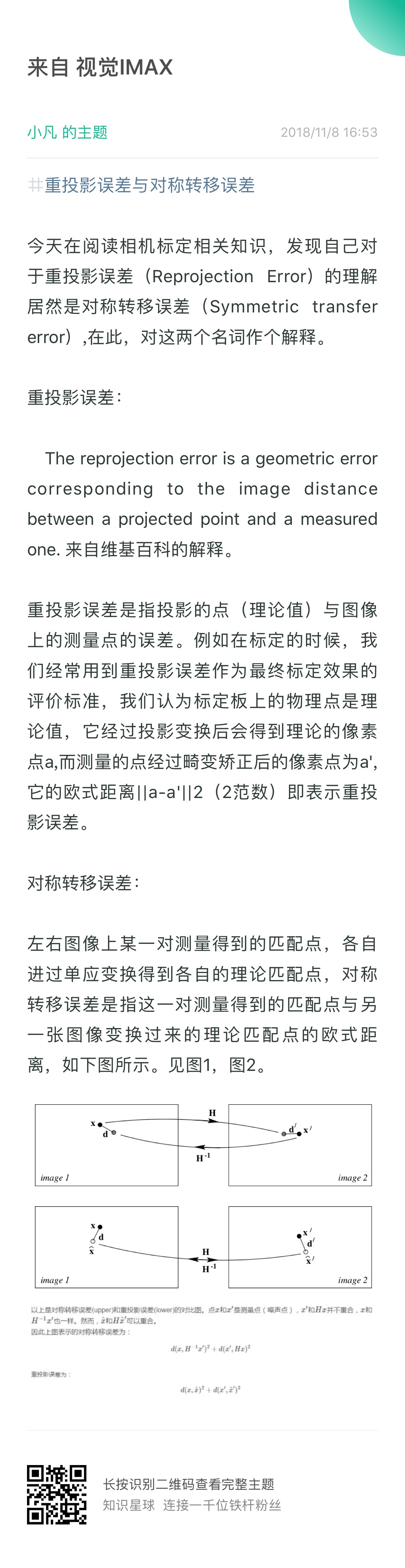展开全文• 该方法以位姿估计初值为中心设置了约束区间, 建立新的罚函数将约束非线性优化转化为无约束非线性优化, 分析像平面重投影误差与约束区间的关系并建立相应的数学模型, 根据模型自动调节约束区间, 基于自适应重投影误差...
• 例如在标定的时候我们经常用到重投影误差作为最终标定效果的评价标准，我们认为标定板上的物理点是理论值，它经过投影变换后会得到理论的像素点a，而测量的点经过畸变矫正后的像素点为a′，它们的欧氏距离||a...
转载 https://blog.csdn.net/sinat_17496535/article/details/51673285

重投影误差

重投影误差是指投影的点（理论值）与图像上的测量点的误差。例如在标定的时候我们经常用到重投影误差作为最终标定效果的评价标准，我们认为标定板上的物理点是理论值，它经过投影变换后会得到理论的像素点a，而测量的点经过畸变矫正后的像素点为a′，它们的欧氏距离||a−a′||2即表示重投影误差。

对称转移误差

左右图像上某一对测量的得到的匹配点，各自经过单应变换得到各自的理论匹配点，对称转移误差是指这一对测量得到的匹配点与另一张图像变换过来的理论匹配点的欧式距离，如下图所示，摘自

Multiple View Geometry in Computer Vision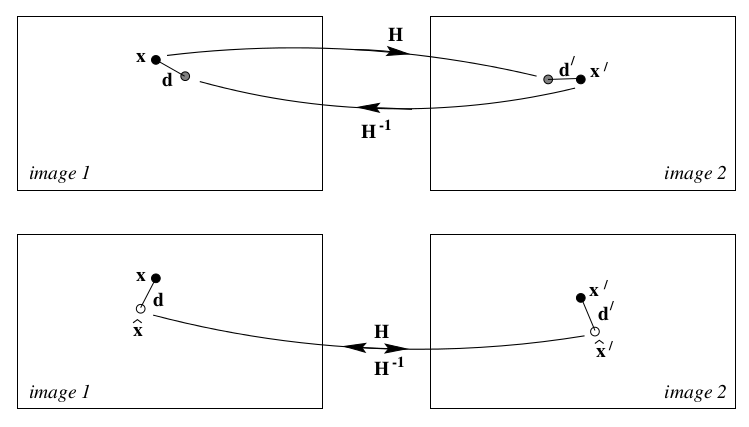以上是对称转移误差以上是对称转移误差(upper)和重投影误差(lower)的对比图。点x和x′是测量点（噪声点），x′和Hx并不重合，x和H−1x′也一样。然而，x̂ 和Hx̂ ′可以重合。
因此上图表示的对称转移误差为：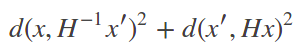重投影误差为：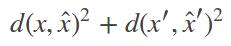展开全文• 这个程序的作用是输入三角化和观测点的地图坐标和位姿，输出计算图像平面重投影误差
• Kalibr源码学习(一): 重投影误差 给自己挖一个大坑, 从标定结果来学习Kalibr的标定源码, 这里基本以KB模型为例, 也就是标定时, kalibr的模型设定为 --model pinhole-equi , 这里以重投影误差开始,希望能坚持; 重投影...
Kalibr源码学习(一): 重投影误差
给自己挖一个大坑, 从标定结果来学习Kalibr的标定源码, 这里基本以KB模型为例, 也就是标定时, kalibr的模型设定为 --model pinhole-equi , 这里以重投影误差开始,希望能坚持;
重投影误差标定结果
首先以重投影误差的txt文档的结果开始,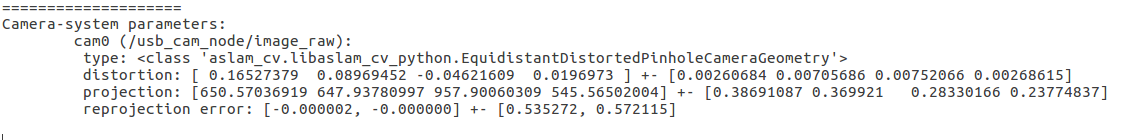可以看到上面的标定结果中, 重投影误差显示为 reprojection error: [-0.000002, -0.000000] +- [0.535272, 0.572115]
这里的两项对应的分别是 reprojection error: [x方向的重投影误差均值, y方向的重投影误差均值] +- [x方向重投影误差方差, y方向重投影误差方差]
其对应的代码片为:
//获取当前检测的角点,重投影坐标, 重投影误差
corners, reprojs, rerrs = getReprojectionErrors(cself, cidx)
if len(rerrs)>0:
//获取重投影误差的均值me和方差se
me, se = getReprojectionErrorStatistics(rerrs)
//打印输出到txt文档中
print >> dest, "\t reprojection error: [%f, %f] +- [%f, %f]" % (me, me, se, se)
print >> dest

这里可以再说以下计算均值和方差的python代码:
在这里插入代码片
def getReprojectionErrorStatistics(all_rerrs):
#传入值是所有的误差 errors, 这里代码里面举出的例子usage是错误的,传入的应该是error,不是重投影
if not len(all_rerrs)>0:
raise RuntimeError("rerrs has invalid dimension")

gc.disable() #append speed up
rerr_matrix=list();
for view_id, view_rerrs in enumerate(all_rerrs):
if view_rerrs is not None: #if cam sees target in this view
for rerr in view_rerrs:
if not (rerr==np.array([None,None])).all(): #if corner was observed
rerr_matrix.append(rerr) #将误差值统一放入到rerr_matrix中
rerr_matrix = np.array(rerr_matrix)
gc.enable()

mean = np.mean(rerr_matrix, 0, dtype=np.float)#计算均值
std = np.std(rerr_matrix, 0, dtype=np.float)#计算方差

return mean, std

重投影误差图像显示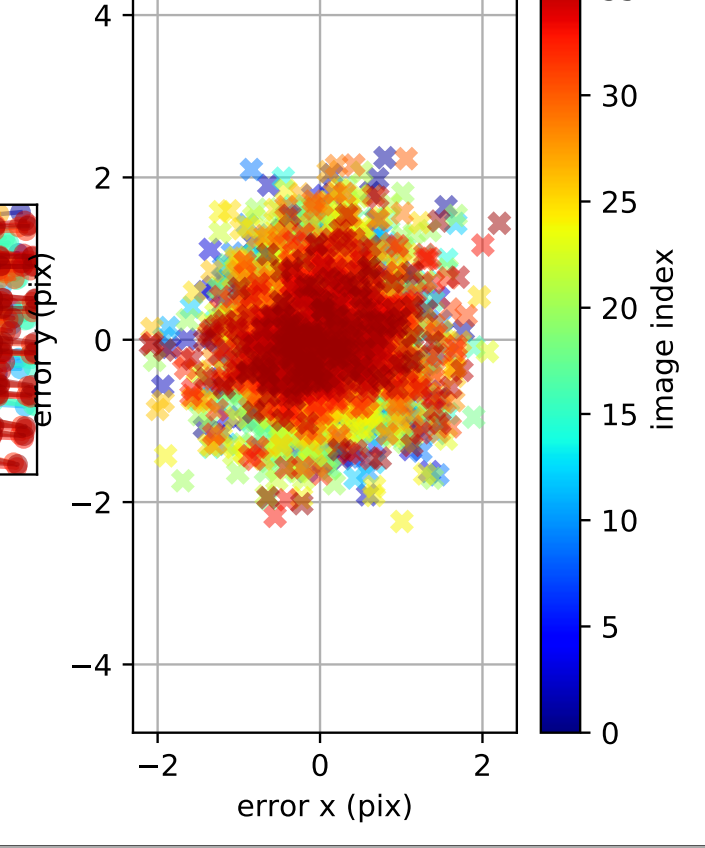这个图对应的代码为:
def plotAllReprojectionErrors(cself, cam_id, fno=1, noShow=False, clearFigure=True, title=""):
# left: observations and projecitons
# right: scatterplot of reprojection errors
all_corners, reprojections, rerrs_xy = getReprojectionErrors(cself, cam_id)
resolution = (cself.cameras[cam_id].geometry.projection().ru(), cself.cameras[cam_id].geometry.projection().rv())

#创建图像
values = np.arange(len(cself.views))/np.double(len(cself.views))
cmap = pl.cm.jet(values,alpha=0.5)

#子图一用来画重投影后的坐标 省略
a=pl.subplot(121)

#子图二用来画重投影误差
sub = pl.subplot(122)
for view_id, rerrs in enumerate(rerrs_xy):
if rerrs is not None: #if this camerea sees the target in this view
color = cmap[view_id,:]
pl.plot(rerrs[:,0], rerrs[:,1], 'x', lw=3, mew=3, color=color)
# 主要是这里,x代表的是重投影的x误差, y表示的是重投影的y值误差, 以 error_x 为横坐标, 以error_y为纵坐标,画图
pl.axis('equal')
pl.grid('on')
pl.xlabel('error x (pix)')
pl.ylabel('error y (pix)')

#省略...

这里重点说一下获取重投影误差的函数, 经过层层调用, 最后计算出的其实是将三维点投影到图像上
//将传入的三维点投影到图像上
template<typename DISTORTION_T>
template<typename DERIVED_P, typename DERIVED_K>
bool PinholeProjection<DISTORTION_T>::euclideanToKeypoint(const Eigen::MatrixBase<DERIVED_P> & p,
const Eigen::MatrixBase<DERIVED_K> & outKeypointConst) const {
EIGEN_STATIC_ASSERT_VECTOR_SPECIFIC_SIZE_OR_DYNAMIC(Eigen::MatrixBase<DERIVED_P>, 3);
EIGEN_STATIC_ASSERT_VECTOR_SPECIFIC_SIZE_OR_DYNAMIC(Eigen::MatrixBase<DERIVED_K>, 2);
Eigen::MatrixBase<DERIVED_K> & outKeypoint = const_cast<Eigen::MatrixBase<DERIVED_K> &>(outKeypointConst);
//shinan note: 转换到归一化相机平面 --> 加畸变 -->转换到像素平面
outKeypoint.derived().resize(2);
double rz = 1.0 / p;
outKeypoint = p * rz;
outKeypoint = p * rz;
//加畸变
_distortion.distort(outKeypoint);

outKeypoint = _fu * outKeypoint + _cu;
outKeypoint = _fv * outKeypoint + _cv;

return isValid(outKeypoint) && p > 0;
}

其中加畸变函数如下:
//将传入的归一化相机坐标系的三维点按照公式加畸变
template<typename DERIVED_Y>
void EquidistantDistortion::distort(const Eigen::MatrixBase<DERIVED_Y> & yconst) const {
EIGEN_STATIC_ASSERT_VECTOR_SPECIFIC_SIZE_OR_DYNAMIC(Eigen::MatrixBase<DERIVED_Y>, 2);
Eigen::MatrixBase<DERIVED_Y> & y = const_cast<Eigen::MatrixBase<DERIVED_Y> &>(yconst);
y.derived().resize(2);
double r, theta, theta2, theta4, theta6, theta8, thetad, scaling;
r = sqrt(y * y + y * y);
theta = atan(r);
theta2 = theta * theta;
theta4 = theta2 * theta2;
theta6 = theta4 * theta2;
theta8 = theta4 * theta4;
thetad = theta* (1 + _k1 * theta2 + _k2 * theta4 + _k3 * theta6 + _k4 * theta8);

scaling = (r > 1e-8) ? thetad / r : 1.0;
y *= scaling;
y *= scaling;
}

总结
这里有一点就是这里的重投影误差的均值很小, 主要的原因可以从上面的彩色图中看出, 重投影误差有正有负,均值会出现互相抵消的状态; 总体来讲是以(-0.000002, -0.000000)为中心, 方差分别是0.535272, 0.572115的正态分布;
所以说,均值不可信,方差大概率更靠谱一些: 如下面标定的很好的结果中:
reprojection error: [0.000000, 0.000001] ± [0.115878, 0.107625]
方差仅为0.1, 其对应的重投影误差的值也都偏小: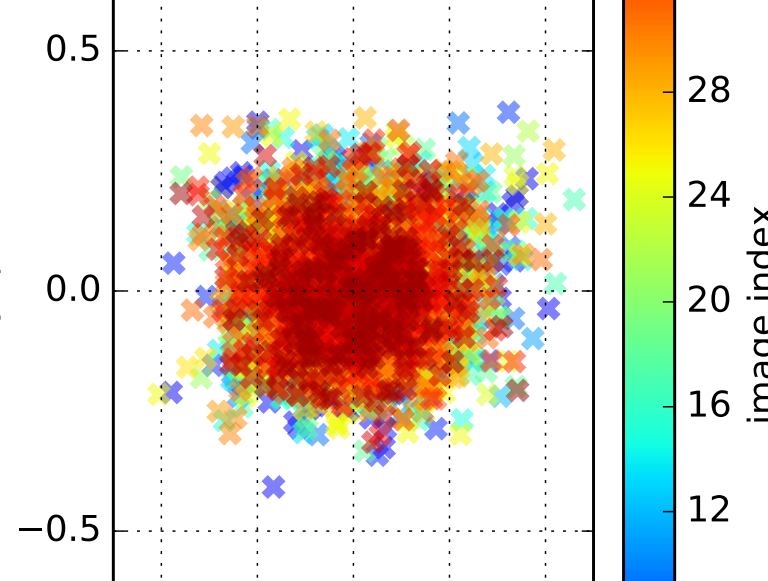根据3*sigma原则, 要想让大部分的重投影误差小于1个像素, 那么其所对应的方差应该是小于0.33.


展开全文鱼眼相机Python
• 为了解决这些问题，我们建议广义重投影误差最小化的两阶段优化方法（TwGREM），其中提出了一种广义的重投影误差框架，以将立体和轮廓提示整合到一个统一的能量中功能。 为了使函数最小化，我们首先在3D体积网格上...研究论文
• 主要是讲解Python利用OpenCV进行相机校正过程中，几个重点参数的分析...准确描述opencv自带的函数，和各个博客、教程里面所说的重投影误差计算的流程进行分析和对比，描述官方的重投影误差和教程实现重投影误差的差别。
OpenCV 校正过程中，calibrateCamera函数的ret和重投影误差的分析
OpenCV对相机进行校正的过程中，校正返回值retval和重投影误差的计算公式表示和分析。OpenCV 校正过程中，calibrateCamera函数的ret和重投影误差的分析一、前言二、calibrateCamera( )函数分析三、projectPoints()函数分析四、具体实验和算法对比五、参考附录

一、前言
本文主要是讲解Python利用OpenCV进行相机校正过程中，几个重点参数的分析。标定的过程就不再一一赘述了，很多博客和网站都在讲解怎么进行标定。本文章主要分析，标定过程中的误差计算公式，和对calibrateCamera( ) 和 projectPoints( ) 两个函数得到的误差不同的原因进行分析。
本文重点分析重投影误差的计算方式。准确描述OpenCV自带的函数，和各个文档，教程里面所说的重投影误差计算的流程进行分析和对比，描述官方的重投影误差和教程实现重投影误差的差别。 我认为OpenCV官方和其他大多数地方的教程，给的重新计算重投影误差的结果，其计算的表达式，也是不准确的。
正确的方法是相机校正的方法输出的重投影误差ret。使用的是RMS均方根误差。另外教程和博客，给的是MSE均方误差
二、calibrateCamera( )函数分析
ret, mtx, dist, rvecs, tvecs = cv2.calibrateCamera(x_world, y_voltage, (224, 224), None, None)

这里，ret表示的是重投影误差；mtx是相机的内参矩阵；dist表述的相机畸变参数；rvecs表示标定棋盘格世界坐标系到相机坐标系的旋转参数：rotation vectors，需要进行罗德里格斯转换；tvecs表示translation vectors，主要是平移参数。
其他几个参数，OpenCV的官网都进行了仔细分析，在这个网站上去仔细的看，每个参数对应的数学公式。https://docs.opencv.org/3.4/dc/dbb/tutorial_py_calibration.html
主要分析ret这个值，也就是retval，这个是重投影误差。
import numpy as np
import cv2 as cv2
import glob
# termination criteria
criteria = (cv2.TERM_CRITERIA_EPS + cv2.TERM_CRITERIA_MAX_ITER, 30, 0.001)
# prepare object points, like (0,0,0), (1,0,0), (2,0,0) ....,(6,5,0)
objp = np.zeros((6*7,3), np.float32)
objp[:,:2] = np.mgrid[0:7,0:6].T.reshape(-1,2)
# Arrays to store object points and image points from all the images.
objpoints = [] # 3d point in real world space
imgpoints = [] # 2d points in image plane.
images = glob.glob('*.jpg')
for fname in images:
gray = cv2.cvtColor(img, cv.COLOR_BGR2GRAY)
# Find the chess board corners
ret, corners = cv2.findChessboardCorners(gray, (7,6), None)
# If found, add object points, image points (after refining them)
if ret == True:
objpoints.append(objp)
corners2 = cv2.cornerSubPix(gray,corners, (11,11), (-1,-1), criteria)
imgpoints.append(corners)
# Draw and display the corners
cv2.drawChessboardCorners(img, (7,6), corners2, ret)
cv2.imshow('img', img)
cv2.waitKey(500)
cv2.destroyAllWindows()

# Calibration, Now that we have our object points and image points, we are ready to go for
# calibration. We can use the function, cv.calibrateCamera() which returns the camera matrix,
# distortion coefficients, rotation and translation vectors etc.
ret, mtx, dist, rvecs, tvecs = cv2.calibrateCamera(objpoints, imgpoints, gray.shape[::-1], None, None)
print("ret:", ret)
print("Camera matrix : \n")
print(mtx)
print("dist : \n")
print(dist)
print("rvecs : \n")
print(rvecs)
print("tvecs : \n")
print(tvecs)


其中，这个ret也就是retval，表示的是重投影误差，是算法自动返回的，其表达式为：
$Reppoints \ x'= k [R|T]X \,.$
其中，Reppoints x’是[Nx2]，表示的图像像素平面的2维坐标点，X是[Nx3]表示的3D世界坐标系下的3维坐标点。也就是棋盘格所构造的3D坐标系的大小。
$ret =repro \_error =\sqrt{\frac{ ||x'-x||_{2}^2}{total\_points}}={\frac{ ||x'-x||_{2}}{\sqrt{total\_points}}}$
这就是cv2.calibrateCamera()返回的值中，ret也就是重投影误差的计算公式。求的是RMS均方根误差，对所有的点，求二范数，然后求平均再开根号，得到RMS误差。
其中，二范数用的是：cv2.norm(data1, data2, cv2.NORM_L2)计算得到:
$norm(x,x')=||x-x'||_2=\sqrt{|| x-x'||_{2}^2}=\sqrt{(x_1-x'_1)^2+...+(x_n-x'_n)^2}$
三、projectPoints()函数分析
imgpoints2, _ = cv.projectPoints(objpoints[i], rvecs[i], tvecs[i], mtx, dist)


原始的计算方法，大多数教程和博客，以及OpenCV文档，给出的重投影误差计算方法。

对相机标定得到的参数，带回原始的投影公式，重新计算从3D世界坐标系投影到2D图像平面坐标系的新的图像像素值，然后计算重投影误差。其中，objpoints表示世界坐标系的坐标，也就是棋盘格的坐标系，rvecs，tvecs，mtx，dist就是相机标定得到的那些参数。
mean_error = 0
for i in range(len(objpoints)):
imgpoints2, _ = cv.projectPoints(objpoints[i], rvecs[i], tvecs[i], mtx, dist)
error = cv.norm(imgpoints[i], imgpoints2, cv.NORM_L2)/len(imgpoints2)
mean_error += error
print( "total error: {}".format(mean_error/len(objpoints)) )

这里计算误差的方式，我们将得到的值和上面相机校正返回的值ret，不一样。因此，需要考虑，将这个官方给定的计算方式，进行重新计算。如果你不在意准确的值，就不用进行优化。
本文是准确的探索，重投影误差的计算方式。按照上面的算法流程，我们可以得到以下的计算重投影误差的公式：
$repro\_error={\frac{ {||x'-x||_{2}}}{total\_points}}$
很明显，均方误差的计算，按照上述的表达式，是不准确的。误差开根号，然后除以点数多少，只能说是每个点的平均欧式误差。MSE误差。

校正之后的重投影误差计算方法或者代码。

因此，需要求得准确的均方根误差，我们应该是先求平方，求平均，在开根号。因此，需要将代码改为：
mean_error = 0
for i in range(len(objpoints)):
imgpoints2, _ = cv.projectPoints(objpoints[i], rvecs[i], tvecs[i], mtx, dist)
error = cv.norm(imgpoints[i], imgpoints2, cv.NORM_L2)
total_error += error*error
print( "rms error: {}".format(np.sqrt(total_error/(len(objpoints)*len(imgpoints2))))

上述的流程才是：
$repro\_error=\sqrt{\frac{(||x-x'||_2^2}{total\_points}}$

与我们的cv2.calibrateCamera()返回的值是一样的。

四、具体实验和算法对比

相机校正返回的值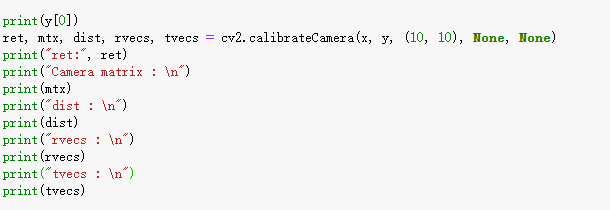函数的返回值
其中的返回的误差项，真实值：ret 为 ret: 0.008683449668964258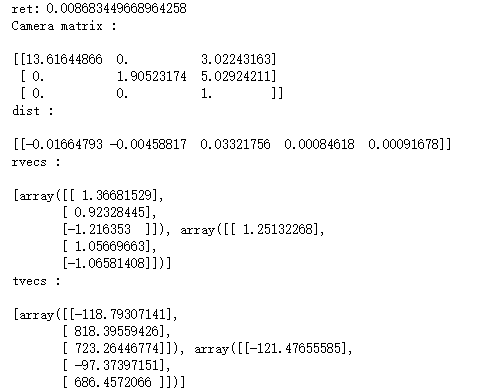使用大多数教程和博客的算法来计算的误差值，是有差别的：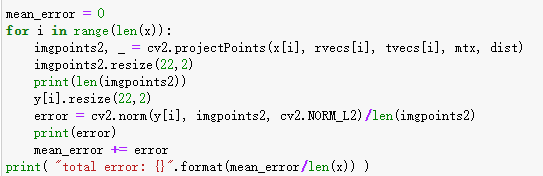参考代码给出的输出误差结果
total error: 0.0018512112930950482

按照自己修正的代码，来进行重投影误差计算：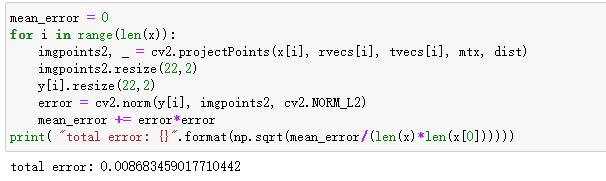输出的重投影误差为：total error: 0.008683459017710442。

因此，和我们相机校正输出的=重投影误差是一样的。
五、参考附录
下面是原始C++函数，计算重投影误差的流程，我上面的公式就是按照这个算法来解释的：
static double computeReprojectionErrors(
const vector<vector<Point3f> >& objectPoints,
const vector<vector<Point2f> >& imagePoints,
const vector<Mat>& rvecs, const vector<Mat>& tvecs,
const Mat& cameraMatrix, const Mat& distCoeffs,
vector<float>& perViewErrors )
{
vector<Point2f> imagePoints2;
int i, totalPoints = 0;
double totalErr = 0, err;
perViewErrors.resize(objectPoints.size());

for( i = 0; i < (int)objectPoints.size(); i++ )
{
projectPoints(Mat(objectPoints[i]), rvecs[i], tvecs[i],
cameraMatrix, distCoeffs, imagePoints2);
err = norm(Mat(imagePoints[i]), Mat(imagePoints2), NORM_L2);
int n = (int)objectPoints[i].size();
perViewErrors[i] = (float)std::sqrt(err*err/n);
totalErr += err*err;
totalPoints += n;
}

return std::sqrt(totalErr/totalPoints);
}

需要准确描述如何计算重投影误差的同学，需要仔细修改你的代码，因为RMS误差来描述的，是需要求平方差，然后求平均，再求平方根。
正确的方法是相机校正的方法输出的重投影误差，而不是大多数，包括OpenCV官方，给出的，都是不一样的，或者是不准确的重投影误差的计算函数。
参考网站：

https://stackoverflow.com/questions/29628445/meaning-of-the-retval-return-value-in-cv2-calibratecamera
https://docs.opencv.org/master/dc/dbb/tutorial_py_calibration.html

`
展开全文• 1. 双目重投影误差项（给的是归一化坐标） //i时刻相机坐标系下的map point坐标 Eigen::Vector3d pts_camera_i = pts_i / inv_dep_i; //i时刻IMU坐标系下的map point坐标 Eigen::Vector3d pts_imu_i = qic * pts_...
• 详细分析一下VINS在初始化的时候对计算出的相机位姿和特征点的3D坐标用ceres进行最小化重投影误差的操作。参考资料：【1】https://www.jianshu.com/p/e5b03cf22c80【2】http://www.ceres-solver.org/index.html【3】...ceres
• 2*6的那个雅克比矩阵是误差对于扰动的小量位姿的偏导数
• https://blog.csdn.net/u010128736/article/details/52875137https://blog.csdn.net/h532600610/article/details/51800488python 角点检测+相机标定+去畸变+重投影误差计算：#coding:utf-8importcv2importnumpy as ...
• mvLevelSigma2; // 图像缩放倍数的平方 // Check reprojection error in second keyframe const float sigmaSquare2 = pKF2->mvLevelSigma2[kp2.octave]; if((errX2*errX2+errY2*errY2)>5.991*sigmaSquare2)
• https://blog.csdn.net/u010128736/article/details/52875137https://blog.csdn.net/h532600610/article/details/51800488python 角点检测+相机标定+去畸变+重投影误差计算：#coding:utf-8importcv2importnumpy as ...
• 在代码中看到了make -j4这样的命令语句，以前自己只用过make mkdir build cd build cmake .. make -j4 用make -j带一个参数，可以把项目在进行并行编译，比如在一台双核的机器上，完全可以用make -j4，让make最多...
• 在SFM（structure from motion）的计算中BA（Bundle Adjustment）作为最后一步优化具有很重要的作用，在近几年兴起的基于图的SLAM（simultaneous localization and mapping）算法里面使用了图优化替代了原来的滤波器......